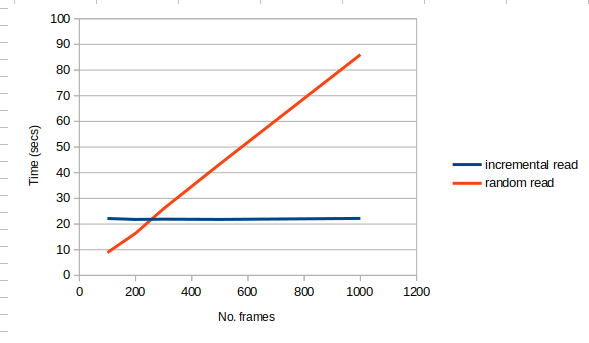Recently, I was working on a program to sample N frames from a source video, and then assign a score to each frame (from 0 to 1) in terms of thumbnail-worthiness. Before long, it became apparent that decoding video frames was one performance bottleneck. In this post, I would look into different ways of reading video frames with OpenCV and then speeding it up with multithreading.

## Reading video frames with OpenCV

Consider that a video is F frame long, we will need to select frame at the sample rate of `S = F/N`. Naiively, one could loop through the video and read every single frame.

``````# naive version
cap = cv2.VideoCapture(video_path)
fno = 0
while success:
if fno % sample_rate == 0:
do_something(img)
``````

However, each call to `cap.read()` would result in unnecessary decoding of frames to be discarded. Performance was intolerable. A better alternative would be to skip through frames and only decode the one that we need. Opencv `grab` and `retrieve` function can be used for this purpose (which are called by `read` function internally).

``````success = cap.grab() # get the next frame
fno = 0
while success:
if fno % sample_rate == 0:
_, img = cap.retrieve()
do_something(img)
success, img = cap.grab()
``````

This simple modification would result in a much needed performance boost. But can we do better? Looking through OpenCV documentation, it’s possible to read from a specific frame by call to `cap.set(cv2.CAP_PROP_POS_FRAMES, fno)`. The above snippet can be re-written as below:

``````total_frames = int(cap.get(cv2.CAP_PROP_FRAME_COUNT))
for fno in range(0, total_frames, sample_rate):
cap.set(cv2.CAP_PROP_POS_FRAMES, fno)
do_something(image)
``````

So which option is faster. It depends on number of frames read relative to total frames. The sparser the read, the faster it is to read with random-seeking method. Since each `cap.set` takes a fixed amount of time to reach certain frame, the latter method’s time cost increases linearly with number of frames to sample. On the other hand, the incremental read method results in a relatively fix amount of time regardless of number of frames to sample.## Sampling video frames faster with multi-threads

If neither of the above methods meet speed requirement, the next natural step would be to parallize frame reading. The idea is simple, instead of one thread reading through the whole video, we would have each worker thread read a continuous segment of the video.

``````fnos = list(range(0, total_frames, sample_rate))

tid = 0
for idx, fno in enumerate(fnos):
``````

A simple worker thread class can be implemented like below, which uses synchronized queue to communicate between main thread and worker threads.

``````class Worker(threading.Thread):

def __init__(self):
self.queue = queue.Queue(maxsize=20)

def decode(self, video_path, fnos, callback):
self.queue.put((video_path, fnos, callback))

def run(self):
"""the run loop to execute frame reading"""
video_path, fnos, on_decode_callback = self.queue.get()
cap = cv2.VideoCapture(video_path)

# set initial frame
cap.set(cv2.CAP_PROP_POS_FRAMES, fnos)
success = cap.grab()

results = []
idx, count = 0, fnos
while success:
if count == fnos[idx]:
success, image = cap.retrieve()
if success:
on_decode_callback(image)
else:
break
idx += 1
if idx >= len(fnos):
break
count += 1
success = cap.grab()

``````

Putting them together:

``````# create and start threads
w = Worker()